• 多项式方程的根
千次阅读
2020-12-17 19:20:51

# 求解一元高次多项式方程的所有实数根的算法

一元高次多项式的形式可以表示为：
f ( x ) = c 0 + c 1 x + c 2 x 2 + c 3 x 3 + . . . + c n x n = 0 f(x) =c _0+c_1x+c_2x^2+c_3x^3+...+c_nx^n=0

一般来说，一元高次多项式方程很难有显示解析解，大多使用数值算法计算方程的根，例如牛顿法、割线法等。但这些算法的缺点是计算得到的解受到迭代初始值的影响，另外在某些特定情况下，迭代也有可能不收敛。对于拥有多个实数根的多项式方程，使用这些迭代算法难以获得方程所有实数根。
本文将介绍一种新的算法，通过递归降次，将高次多项式方程的求解问题逐步转化为可以解析求解的一元二次方程，进而求解出多项式的所有实数根。

## 原理

首先，为了求解多项式方程的所有实根，我们需要对多项式函数进行分段。使用 x 1 , x 2 , x 3 , . . . , x k x_1,x_2,x_3,...,x_k 这一系列的点将实数轴分成若干区间段，以使得在每一个区间段上函数f(x)都是单调的。
当我们获得了这一系列的分隔点后，例如上述k个分隔点，将实数轴分为k+1个区间段，（包括 ( − ∞ , x 1 ] (-\infin,x_1] [ x k , + ∞ ) [x_k,+\infin) 两个区间）。在每一个区间中函数都是单调的。对于区间 [ x i , x i + 1 ] [x_i,x_{i+1}] ，如果 f ( x i ) f(x_i) f ( x i + 1 ) f(x_{i+1}) 符号相反，由于函数在区间中是单调的，在该区间中必然有且仅有一个实根。这时只要我们在该区间中使用最简单的二分法就能得到该区间中的或者一个实根。
当然，如果 f ( x i ) f(x_i) f ( x i + 1 ) f(x_{i+1}) 符号相同，由于函数单调，在该区间中必然不存在方程的实根。
对于 ( − ∞ , x 1 ] (-\infin,x_1] 这个区间，可以先算出 f ( x 1 ) f(x_1) f ( x 1 − 1 ) f(x_1-1) ，如果 f ( x 1 − 1 ) f(x_1-1) f ( x 1 ) f(x_1) 同号，且 f ( x 1 − 1 ) f(x_1-1) f ( x 1 ) f(x_1) 更远离0，由于函数的单调性，在这个区间上必然不存在实根。如果不是这种情况，我们就需要依次计算 f ( x 1 − 1 ) , f ( x 1 − 2 ) , f ( x 1 − 4 ) , . . . , f ( x 1 − 2 m ) , . . . f(x_1-1),f(x_1-2),f(x_1-4),...,f(x_1-2^m),... ，直到找到一个与 f ( x 1 ) f(x_1) 符号相反的值，例如 x 1 − 2 m x_1-2^m ，然后再在区间 [ x 1 − 2 m , x 1 ] [x_1-2^m,x_1] 上使用二分法求解出相应的实数根。
对于区间 [ x k , + ∞ ) [x_k,+\infin) 也使用一样的处理方法，只是将上面的减号变成加号即可。
使用以上的方法，我们发现，只要我们能够找到这一系列的分隔点，使得分隔出的每一个区间上多项式函数都是单调的，那么这个多项式方程求解的问题就解决了。
那么问题就转换为如何找到这一系列的分隔点。对于多项式函数，函数的一阶导数等于0的点，正好就是这样的分隔点。我们只要求解出函数f(x)的所有一阶导数等于0的点即可。即问题转化为求解方程 f ′ ( x ) = 0 f^{'}(x)=0 也就是
f ′ ( x ) = c 1 + 2 c 2 x + 3 c 3 x 2 + 4 c 4 x 3 + . . . + n c n x n − 1 = 0 f^{'}(x) =c _1+2c_2x+3c_3x^2+4c_4x^3+...+nc_nx^{n-1}=0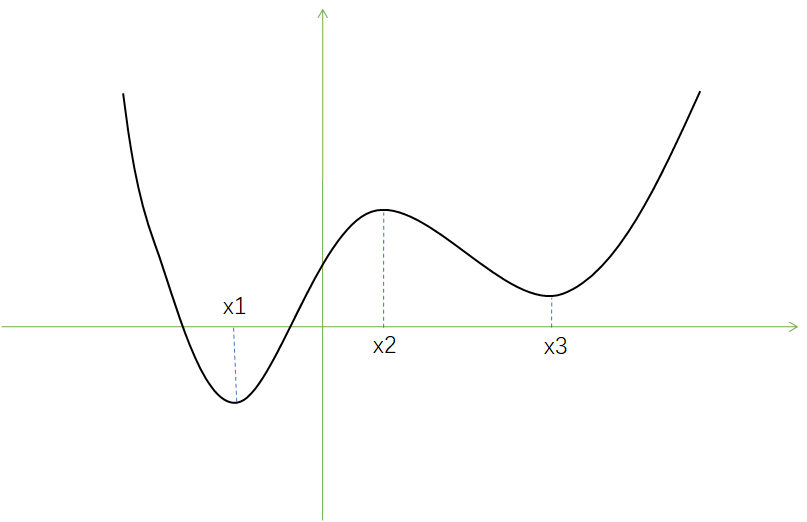经过以上的处理，我们将求解f(x)=0方程的问题转换成了求解其一阶导函数等于0的方程求解问题，这样使得我们求解的方程的次数下降了一阶。
使用这种方法，递归处理，我们最终一定能够将问题逐步转换为可以解析求解的一元二次方程。
可以看到，以上求解的过程完全不需要外部指定任何初始值以及其他变量，只需要输入方程每一项的系数，就可以求解出所有了方程实根。

## 代码实现（C#）

    /// <summary>
/// 求解单一未知数多项式方程，c0+c1*x^1+c2*x^2+c3*x^3+...+cn*x^n=0
/// </summary>
public class SingleUnknownPolynomialSolver
{
/// <summary>
/// 传入系数数组，构建实例
/// </summary>
/// <param name="coefs">方程系数：c0、c1、c2、c3、...、cn</param>
public SingleUnknownPolynomialSolver(params double[] coefs)
{
if (coefs == null) _c = null;
int cLength = 0;
for (int i = 0; i < coefs.Length; i++)
{
if (coefs[coefs.Length - 1 - i] == 0) continue;
cLength = coefs.Length - i;
break;
}
_c = coefs.Take(cLength).ToArray();
}

private double[] _c;
private Mathematics.ApproxEqual _approx = new Mathematics.ApproxEqual();

/// <summary>
/// 获取或设置近似相等比较器，近似相等比较器不可以为null，如果设置null则使用默认的近似相等比较器
/// </summary>
public Mathematics.ApproxEqual ApproxEqual
{
get => _approx;
set
{
if (value == null) _approx = new Mathematics.ApproxEqual();
else _approx = value;
}
}

/// <summary>
/// 求解方程的所有实数解
/// </summary>
/// <returns>返回所有实数解，无解返回空数组，无穷解返回null</returns>
public double[] Solve()
{
if (_c == null || _c.Length == 0) return null;
if (_c.Length == 1) return new double[] { };
if (_c.Length == 2) return new double[] { -_c / _c };
if (_c.Length == 3)
{
double delta = _c * _c - 4 * _c * _c;
if (delta < 0) return new double[] { };
double sqrtDelta = Math.Sqrt(delta);
if (_approx.IsEqual(sqrtDelta / _c, 0)) return new double[] { -_c / _c / 2 };
return new double[] { (-_c + sqrtDelta) / _c / 2, (-_c - sqrtDelta) / _c / 2 };
}
var partialSolver = PartialSolver();
var partialSolutions = partialSolver.Solve();
if (partialSolutions.Length == 0)
{
double? x_down = OppositePoint(0, false);
if (x_down != null)
{
double? solution = SolvePoint(x_down ?? 0, 0);
if (solution != null) return new double[] { solution ?? 0 };
else return new double[] { };
}
double? x_up = OppositePoint(0, true);
if (x_up != null)
{
double? solution = SolvePoint(0, x_up ?? 0);
if (solution != null) return new double[] { solution ?? 0 };
else return new double[] { };
}
return new double[] { };
}
List<double> solutionList = new List<double>();
var partialSolutionList = partialSolutions.ToList();
partialSolutionList.Sort();
partialSolutions = partialSolutionList.ToArray();
double? x_d = OppositePoint(partialSolutions.First(), false);
if (x_d != null)
{
double? solution = SolvePoint(x_d ?? 0, partialSolutions.First());
if (solution != null) solutionList.Add(solution ?? 0);
}
double? x_u = OppositePoint(partialSolutions.Last(), true);
if (x_u != null)
{
double? solution = SolvePoint(partialSolutions.Last(), x_u ?? 0);
if (solution != null) solutionList.Add(solution ?? 0);
}
if (partialSolutions.Length >= 2)
{
for (int i = 1; i < partialSolutions.Length; i++)
{
double? solution = SolvePoint(partialSolutions[i - 1], partialSolutions[i]);
if (solution != null) solutionList.Add(solution ?? 0);
}
}
solutionList.Sort();
for (int i = 1; i < solutionList.Count; i++)
{
if (_approx.IsEqual(solutionList[i - 1], solutionList[i]))
{
solutionList.RemoveAt(i);
i--;
}
}
return solutionList.ToArray();
}

/// <summary>
/// 计算函数值
/// </summary>
/// <param name="x"></param>
/// <returns></returns>
private double CalcValue(double x)
{
double y = 0;
for (int i = 0; i < _c.Length; i++)
{
y += _c[i] * Math.Pow(x, i);
}
return y;
}

/// <summary>
/// 一阶导函数方程
/// </summary>
/// <returns></returns>
private SingleUnknownPolynomialSolver PartialSolver()
{
double[] coefs = new double[_c.Length - 1];
for (int i = 1; i < _c.Length; i++)
{
coefs[i - 1] = _c[i] * i;
}
SingleUnknownPolynomialSolver solver = new SingleUnknownPolynomialSolver(coefs);
solver.ApproxEqual = _approx;
return solver;
}

/// <summary>
/// 求解x点函数值符号相反的点，要求指定的方向上函数是单调的
/// </summary>
/// <param name="x"></param>
/// <param name="upper">向上搜索还是向下搜索</param>
/// <returns></returns>
private double? OppositePoint(double x, bool upper = false)
{
double s = upper ? 1 : -1;
double y = CalcValue(x);
if (y == 0) return x;
double m = 1;
while (m < double.MaxValue)
{
double xp = x + m * s;
double yp = CalcValue(xp);
if (yp == 0) return xp;
if (yp == y) return null;
if (yp > y && y > 0) return null;
if (yp < y && y < 0) return null;
if (yp > 0 && y < 0) return xp;
if (xp < 0 && y > 0) return xp;
m = m * 2;
}
return null;
}

/// <summary>
/// 求解两个点中间的方程解，要求在两点之间函数单调
/// </summary>
/// <param name="xl">下方点</param>
/// <param name="xu">上方点</param>
/// <returns></returns>
private double? SolvePoint(double xl, double xu)
{
if (xl > xu) return null;
if (xl == xu)
{
if (CalcValue(xl) == 0) return xl;
else return null;
}
double l = xl;
double u = xu;
while (!_approx.IsEqual(l, u))
{
double yl = CalcValue(l);
if (yl == 0) return l;
double yu = CalcValue(u);
if (yu == 0) return u;
if (yl > 0 && yu > 0) return null;
if (yl < 0 && yu < 0) return null;
double m = (l + u) / 2;
double ym = CalcValue(m);
if (ym == 0) return m;
if (ym > 0 && yl < 0) u = m;
else if (ym > 0 && yl > 0) l = m;
else if (ym < 0 && yl < 0) l = m;
else u = m;
}
return (u + l) / 2;
}

}



## 关于代码的一些说明

1、算法的核心在于Solve方法中，采用嵌套调用的形式。OppositePoint方法用于处理边界区间搜索异号函数值点，SolvePoint方法用于求解在指定的区间上使用二分法求出区间上的方程根。
2、在构造函数中对输入的系数数组进行了预处理，以保证多项式的最高次项的系数不为0。
3、上述代码中没有给出Mathematics.ApproxEqual类的具体定义。实际上ApproxEqual是一个近似相等比较器，它的IsEqual方法可以比较两个实数在指定的精度和算法下是否近似相等。在实际的应用中，读者可以根据自己的需要定义自己的近似相等比较器的具体算法和参数。算法
更多相关内容
• 幂法求多项式方程的模最大matlab实现 要求:利用maab编写通用子程序,利用幂法求多项式方程的解: 思想 首先要将多项式转化成矩阵形式通过老师上课讲的内容将上述多项式转化成为如家格式的矩阵 此矩阵的特征值就是...
• ## 多项式方程求根

千次阅读 2021-05-25 09:55:42
的特征值就是的. 使用Eigen进行多项式的求解： #include <iostream> #include <Eigen/Core> // 稠密矩阵的代数运算（逆，特征值等） #include <Eigen/Dense> using namespace std; int main...

参考别人的博客,顺便借鉴过来作个笔记.

一.使用多项式的伴随矩阵进行求解

多项式:

多项式的伴随矩阵:

的特征值就是的根.

其中是个方阵,是个列向量,是个常量,其中是矩阵的特征向量,是矩阵的特征值.

使用Eigen进行多项式的求解：

#include <iostream>
#include <Eigen/Core>
// 稠密矩阵的代数运算（逆，特征值等）
#include <Eigen/Dense>
using namespace std;

int main( int argc, char** argv )
{

Eigen::Matrix<double, 4, 4> matrix_44;
//复数动态矩阵
//P(x) = x^4 + 2*x^3 +3*x^2 +4*x + 5;
Eigen::Matrix<complex<double>, Eigen::Dynamic, Eigen::Dynamic> matrix_eigenvalues;
//同样测试 12345
matrix_44 <<                 0, 0, 0, -5,
1, 0, 0, -4,
0, 1, 0, -3,
0, 0, 1, -2;

std::cout<<"matrix_44: "<<std::endl<<matrix_44<<std::endl<<std::endl;

matrix_eigenvalues = matrix_44.eigenvalues();
//std::cout<<"matrix_44.eigenvalues: "<<std::endl<<matrix_44.eigenvalues()<<std::endl;
std::cout<<"matrix_eigenvalues: "<<std::endl<<matrix_eigenvalues<<std::endl;
return 0;
}



或者使用Eigen多项式

#include<iostream>
#include <Eigen/Eigen>
#include <unsupported/Eigen/Polynomials>
using namespace std;

int main()
{
Eigen::PolynomialSolver<double,Eigen::Dynamic> solver; //多项式求根
Eigen::VectorXd coeff(5);
coeff = 5;
coeff = 4;
coeff = 3;
coeff = 2;
coeff = 1;
solver.compute(coeff);  //compute()计算多项式复数根
const Eigen::PolynomialSolver<double,Eigen::Dynamic>::RootsType& r = solver.roots();  //roots()返回多项式复数根
std::vector<double> real_root;
for (int i = 0; i< r.rows();++i)
{
if (r[i].imag() != 0.0)
{
continue;
}
real_root.push_back(r[i].real());
}

for(int i=0;i<real_root.size();++i)
{
cout<<"多项式方程的实根:"<<real_root[i]<<std::endl;
}
return 0;

}

参看链接:

https://blog.csdn.net/fb_941219/article/details/102984587

http://eigen.tuxfamily.org/dox-devel/unsupported/classEigen_1_1PolynomialSolverBase.html#a07bcd5339be5eacdf7e566d07d81bedb

展开全文• 例如：二次函数：f(x) = 10x^2 - 14x + 22，依次求解x=1，2，3，4，5，6时函数表达式所对应的函数值。 import numpy as np #f(x) = 10*x^2 - 14x + 22 p = np.array([10,-14,22])#这里存放的是系数 ...

# 例如：二次函数：f(x) = 10x^2 - 14x + 22，依次求解x=1，2，3，4，5，6时函数表达式所对应的函数值。

import numpy as np
#f(x) = 10*x^2 - 14x + 22
p = np.array([10,-14,22])#这里存放的是系数
x = [1,2,3,4,5,6]
y=np.polyval(p,x)#这里的列表y依次存放x=1、x=2、x=3、x=4、x=5、x=6 所对应的y的值
print(y)


结果如下：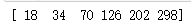# 例如：三次函数：f(x) = 10x^3 - 14x ^2 + 22*x + 5，依次求解x=1，2，3时函数表达式所对应的函数值。

import numpy as np
#f(x) = 10*x^3 - 14*x ^2 + 22*x + 5
p = np.array([10,-14,22,5])#这里存放的是系数
x = [1,2,3]
y=np.polyval(p,x)#这里的列表y依次存放x=1、x=2、x=3所对应的y的值
print(y)


结果如下：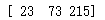# 例如：四次函数：f(x) = 10x^4 - 14x ^3 + 22x ^2 + 6x + 99，依次求解x=1，2，3，4，5，6，7，8，9，10时函数表达式所对应的函数值。

import numpy as np
#f(x) = 10*x^4 - 14*x ^3 + 22*x ^2 + 6*x + 99
p = np.array([10,-14,22,6,99])#这里存放的是系数
x = [1,2,3,4,5,6,7,8,9,10]
y=np.polyval(p,x)#这里的列表y依次存放x=1、x=2、x=3、x=4、x=5、x=6、x=7、x=8、x=9、x=10所对应的y的值
print(y)


结果如下：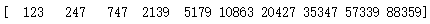展开全文python
• 本研究提出了一种求解多项式方程的新方法。 描述了基于最近开发的用于求解三次方程的新方法的两种求解四次方程的技术。 高阶多项式方程是通过... 建议在规定的条件下，提出的方法提供了寻找多项式方程根的有效技术。
• 方程 inv - 逆矩阵 roots - 多项式newton - 牛顿迭代法解非线性方程
• 再论实数型多项式方程进行根式求解的条件，罗林，，这篇文章专题研究了由两个多项式函数的代数和所定义方程中，因两个函数的常数项在非线性空间作用下相互抵消提取的一次公因式与这
• 运用多项式方程根的性质理论及著名的 牛顿公式, 解决 了牛 领公 式用于多项式方程 时迭 代初值的选取, 并求出多项式方程的所有实根 。 同时给 出了算例。
• 二等分法的一个优点是可以用于在负x轴处查找。 但是，它只能用于在一定范围内找到多项式的一个matlab
• 在PnL方法求相机位姿，Point—to—Line方法的点云匹配问题中，会将其中某一个参数的求解建模成求一个多项式。如下式所示： 要将矩阵的特征值和多项式联系起来，我们可以假设此时多项式就是矩阵 也...

在PnL方法求相机位姿，Point—to—Line方法的点云匹配问题中，会将其中某一个参数的求解建模成求一个多项式的根。如下式所示：

(1)

那么求解这样的一个多项式的根应该怎么做呢？参考文献1告诉我们可以利用矩阵特征值方法来求解，下面就简单的记录一下这个方法。

(2)

而矩阵特征值的定义为：

(3)

要将矩阵A的特征值和多项式P(x) 的根联系起来，我们可以假设此时多项式P(x)的根就是矩阵 A阵的特征值，因此有：

(4)

也就是说应该要有一个合理的矩阵A和特征向量y 能将(2)式等价为(4)式。

此时如果令：，就很容易得到矩阵A了。

(5)

那么接下来就用简单的代码验证下。

#include <iostream>
#include <Eigen/Core>
#include <Eigen/Geometry>
#include <Eigen/Dense>
#include<unsupported/Eigen/Polynomials>
using namespace std;

int main()
{
//setting coefficient of polynomial
// 9x^4+ 34x^3 + x^2 -100x +201 =0

Eigen::VectorXf coeffs(5);
coeffs(4) = 9;
coeffs(3) = 34;
coeffs(2) = 0;
coeffs(1) = 0;
coeffs(0) = 9;
Eigen::Matrix4f A;
A << -coeffs(3)/coeffs(4),-coeffs(2)/coeffs(4),-coeffs(1)/coeffs(4),-coeffs(0)/coeffs(4),
1,                    0,                    0,                 0,
0,                    1,                    0,                 0,
0,                    0,                    1,                 0;

// Slove

Eigen::EigenSolver<Eigen::MatrixXf> ES(A);
std::cout << "Roots:\n" << ES.eigenvalues().transpose() << std::endl;

// // Check results
Eigen::PolynomialSolver<float,4> solve;
solve.compute(coeffs);
cout << "Eigen Method:\n" << solve.roots().transpose() << endl;
return 0;
}

结果如下：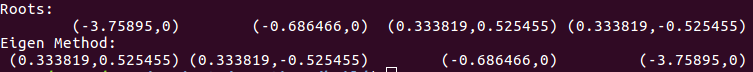同步更新在：

https://zhuanlan.zhihu.com/p/363946630

展开全文自动驾驶
• Matlab多项式运算与方程对各种多项式运算给出实列和方法
• ## 多项式的求根方法

万次阅读 2020-11-15 17:09:09
函数f(x)=(an)xⁿ +....+ (a4)x⁴ + (a3)x³ + (a2)x² + (a1)x¹ + a0是一个多项式函数，一般情况下直接求f(x)=0的不是很方便。所以存在一个试的技巧。 二 多项式函数求的试法 1. 如果a0 = 0，则f(0) =...数学
• 多项式方程的实或复根。 C++: double solvePoly ( InputArray coeffs, OutputArray roots, int maxIters=300 ) 参数说明： coeffs - 多项式系数数组， coeffs 是从常数项到最高阶。 roots - 输出(复数)...
• matlab 求解多项式 四种方法 方法一 %多项式函数roots p=[1,-3,1];%建立多项式系数向量p x=roots(p)%求多项式 x=-5:0.1:5;%步长为0.1 y1=x.*x-3*x+1;%构建一个函数 y2=zeros(size(x));%产生一个与x同...
• 2017-10-09 回答用matlab解方程的三个实例1、对于多项式p(x)=x3-6x2-72x-27，求多项式p(x)=0的，可用多项式函数roots(p)，其中p为多项式系数向量，即>>p =p =1.00 -6.00 -72.00 -27.00p是多项式的matlab...
• 如果寻找复杂的，这种方法可能是有利的，因为即使以前的迭代是真实的，任何迭代也可能是复杂的。 运行代码的过程： 按 F5 或 RUN，然后在命令窗口中将显示一条消息-“n 阶多项式函数的类型为：aX^n+aX^(n-...matlab
• matlab 中方程求解的基本命令 roots(p):求多项式方程,其中 p 是多项式系数按降幂排列所形成的向量。 solve(fun):求方程 fun=0 的符号解,如果不能求得精确......多项式加减运算:Matlab没有提供专门进行多项式 加...
• 先说明一下多项式方程的特点，对于n次多项式方程，实数的可能情况包括（此处只说明实数）： 1、有n个不同实； 2、的数量小于n，大于0，即有多个相同实； 3、无实数，即方程所表示的曲线与X轴无交点...opencv
• 最方便，最实用的多项式运算器，方程组求解器，希望对大家有用。
• MATLAB牛顿（Newton）迭代法和割线法求解方程 MATLAB牛顿（Newton）迭代法和割线法求解方程
• python在进行多项式时，当多项式阶数增大，使用Numpy.roots求会导致求结果不准确，甚至产生错误的复数 如下求解9阶多项式 import numpy as np distort_poly = np.poly1d([D, 0, D, 0, D, 0, ...python 开发语言 后端
• Matlab 多项式求解 分享一下通过多种不同的方法计算多项式。 数值 使用代换法求 特定区间内的 符号 数值 roots函数用于计算系数向量表示的单变量多项式。 例如，创建...Matlab
• 在matlab平台下，适当选择初始点，通过割线法，求解方程，不需要像牛顿法一样求导，对计算的要求降低
• 在Matlab中新建脚本，在脚本中输入 p = [1 -6 3 26 -24]; format long; roots§ 点击运行，可得如下答案Matlab
• 1.创建多项式 import numpy as np # f(x) = x^3 - 2x + 1 a = np.array([1, 0, -2, 1])#从最高次方开始排 fx = np.poly1d(a) #poly1d([ 1, 0, -2, 1]) type(fx) #numpy.lib.polynomial.poly1d fx(1)#把1代入求解 ...python numpy
• 文章目录MATLAB的roots函数GSL库的gsl_poly_complex_solve求解器参考来源 ...包含带有非负指数的单一变量的多项式方程。 对方程 x 4 −1=0 求解。 创建一个向量，用它来代表多项式，然后计算多项式的
• R中帮助文件对“ployroot”的解释是“Find zeros of a real or complex polynomial”，翻译过来是“多项式系数递增的向量”。可能有些抽象，下面用几个例子帮助大家理解ployroot函数的用法。 我们设要求解的方程为x2...r语言 开发语言
• 多项式方程组解的有理单变量表示及其应用【摘要】：多项式方程组求解是一个古老、经典但却依旧充满活力的问题.它在数学领域理论研究及工程领域实践应用中都起着至关重要的作用.多项式方程组根据解的个数可分为零维......